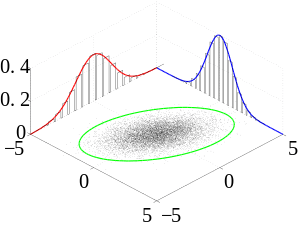# Joint probability distributionMany sample observations (black) are shown from a joint probability distribution. The marginal densities are shown as well.

In the study of probability, given at least two random variables X, Y, ..., that are defined on a probability space, the joint probability distribution for X, Y, ... is a probability distribution that gives the probability that each of X, Y, ... falls in any particular range or discrete set of values specified for that variable. In the case of only two random variables, this is called a bivariate distribution, but the concept generalizes to any number of random variables, giving a multivariate distribution.

The joint probability distribution can be expressed either in terms of a joint cumulative distribution function or in terms of a joint probability density function (in the case of continuous variables) or joint probability mass function (in the case of discrete variables). These in turn can be used to find two other types of distributions: the marginal distribution giving the probabilities for any one of the variables with no reference to any specific ranges of values for the other variables, and the conditional probability distribution giving the probabilities for any subset of the variables conditional on particular values of the remaining variables.

## Examples

### Coin Flips

Consider the flip of two fair coins; let A and B be discrete random variables associated with the outcomes first and second coin flips respectively. If a coin displays "heads" then associated random variable is 1, and is 0 otherwise. The joint probability mass function of A and B defines probabilities for each pair of outcomes. All possible outcomes areSince each outcome is equally likely the joint probability mass function becomeswhen. Since the coin flips are independent, the joint probability mass function is the product of the marginals:.

In general, each coin flip is a Bernoulli trial and the sequence of flips follows a Bernoulli distribution.

### Dice Rolls

Consider the roll of a fair die and let A = 1 if the number is even (i.e. 2, 4, or 6) and A = 0 otherwise. Furthermore, let B = 1 if the number is prime (i.e. 2, 3, or 5) and B = 0 otherwise.

1 2 3 4 5 6
A 0 1 0 1 0 1
B 0 1 1 0 1 0

Then, the joint distribution of A and B, expressed as a probability mass function, isThese probabilities necessarily sum to 1, since the probability of some combination of A and B occurring is 1.

## Density function or mass function

### Discrete case

The joint probability mass function of two discrete random variablesis:whereis the probability ofgiven that.

The generalization of the preceding two variable case is the joint probability distribution ofdiscrete random variableswhich is:This identity is known as the chain rule of probability.

Since these are probabilities, we have in the two-variable casewhich generalizes fordiscrete random variablesto### Continuous case

The joint probability density function fX,Y(x, y) for continuous random variables is equal to:…where fY|X(y|x) and fX|Y(x|y) give the conditional distributions of Y given X = x and of X given Y = y respectively, and fX(x) and fY(y) give the marginal distributions for X and Y respectively.

Again, since these are probability distributions, one has### Mixed case

The "mixed joint density" may be defined where one random variable X is continuous and the other random variable Y is discrete, or vice versa, as:One example of a situation in which one may wish to find the cumulative distribution of one random variable which is continuous and another random variable which is discrete arises when one wishes to use a logistic regression in predicting the probability of a binary outcome Y conditional on the value of a continuously distributed outcome X. One must use the "mixed" joint density when finding the cumulative distribution of this binary outcome because the input variables (X, Y) were initially defined in such a way that one could not collectively assign it either a probability density function or a probability mass function. Formally, fX,Y(x, y) is the probability density function of (X, Y) with respect to the product measure on the respective supports of X and Y. Either of these two decompositions can then be used to recover the joint cumulative distribution function:The definition generalizes to a mixture of arbitrary numbers of discrete and continuous random variables.

### Joint distribution for independent variables

Two discrete random variablesandare independent if the joint probability mass function satisfiesfor all x and y.

Similarly, two absolutely continuous random variables are independent iffor all x and y. This means that acquiring any information about the value of one or more of the random variables leads to a conditional distribution of any other variable that is identical to its unconditional (marginal) distribution; thus no variable provides any information about any other variable.

### Joint distribution for conditionally dependent variables

If a subsetof the variablesis conditionally dependent given another subsetof these variables, then the joint distributionis equal to. Therefore, it can be efficiently represented by the lower-dimensional probability distributionsand. Such conditional independence relations can be represented with a Bayesian network or copula functions.

### Cumulative distribution

The joint probability distribution for a pair of random variables can be expressed in terms of their cumulative distribution function## Important named distributions

Named joint distributions that arise frequently in statistics include the multivariate normal distribution, the multivariate stable distribution, the multinomial distribution, the negative multinomial distribution, the multivariate hypergeometric distribution, and the elliptical distribution.